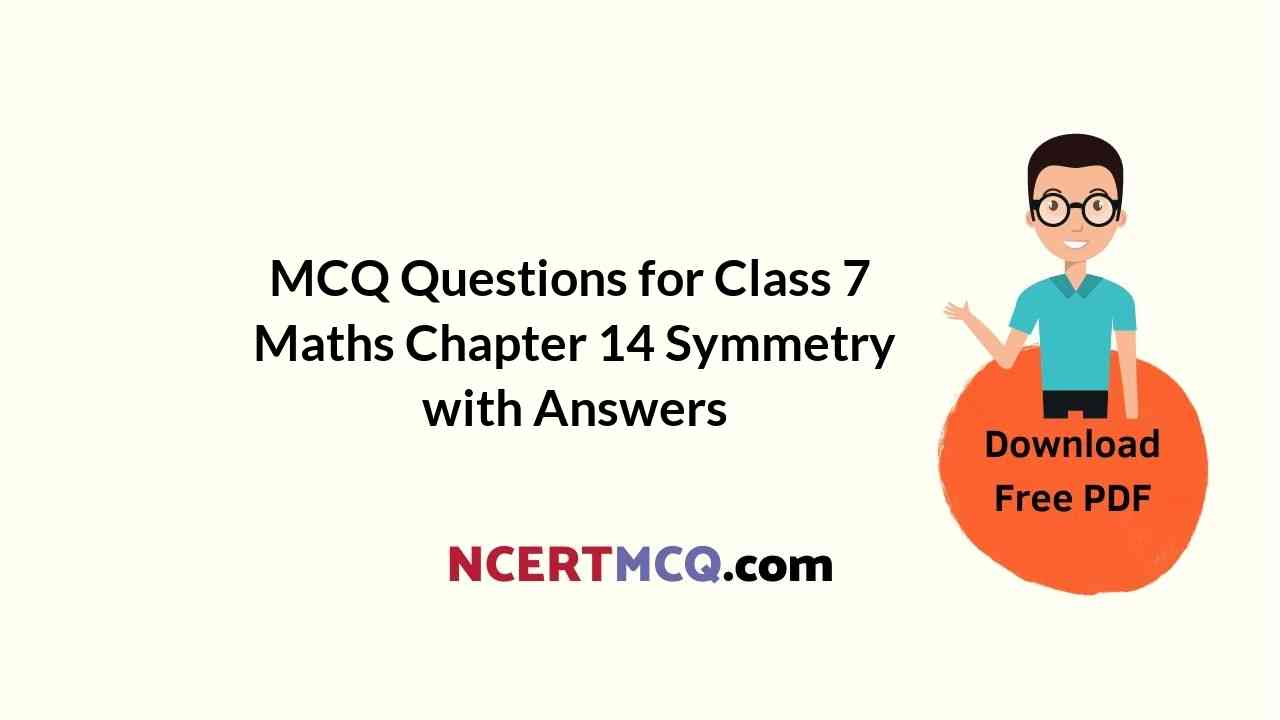Check the below NCERT MCQ Questions for Class 7 Maths Chapter 14 Symmetry with Answers Pdf free download. MCQ Questions for Class 7 Maths with Answers were prepared based on the latest exam pattern. We have provided Symmetry Class 7 Maths MCQs Questions with Answers to help students understand the concept very well. https://ncertmcq.com/mcq-questions-for-class-7-maths-with-answers/

Students can also refer to NCERT Solutions for Class 7 Maths Chapter 14 Symmetry for better exam preparation and score more marks.

## Symmetry Class 7 MCQs Questions with Answers

Symmetry Class 7 MCQ Chapter 14  Question 1.
How many lines of symmetry are there in an equilateral triangle?
(a) 1
(b) 2
(c) 3
(d) 4

MCQ On Symmetry For Class 7 Chapter 14  Question 2.
How many lines of symmetry are there in a square?
(a) 1
(b) 2
(c) 3
(d) 4

Class 7 Symmetry MCQ Chapter 14  Question 3.
How many lines of symmetry are there in a rectangle?
(a) 1
(b) 2
(c) 3
(d) 4

Symmetry MCQ Class 7 Chapter 14  Question 4.
How many lines of symmetry are there in a regular pentagon?
(a) 1
(b) 2
(c) 3
(d) 5

MCQ Questions On Symmetry For Class 7 Chapter 14  Question 5.
How many lines of symmetry are there in a regular hexagon?
(a) 2
(b) 4
(c) 6
(d) 3

MCQ Of Symmetry Class 7 Chapter 14  Question 6.
How many lines of symmetry are there in the following figure?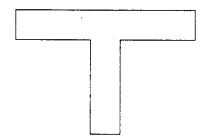(a) 1
(b) 2
(c) 3
(d) 4

MCQ Questions For Class 7 Maths Symmetry Chapter 14  Question 7.
How many lines of symmetry are there in the following figure?(a) 1
(b) 2
(c) 3
(d) 4

Class 7 Maths Chapter 14 MCQ  Question 8.
How many lines of symmetry are there in the following figure?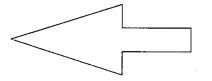(a) 1
(b) 2
(c) 3
(d) 4

Class 7 Maths Chapter 14 MCQ With Answers Question 9.
How many lines of symmetry are there in the following figure?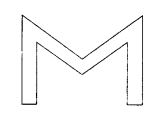(a) 4
(b) 3
(c) 2
(d) 1

Symmetry Multiple Choice Questions Chapter 14  Question 10.
How many lines of symmetry are there in the following figure?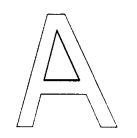(a) 2
(b) 1
(c) 4
(d) 3

Class 7 Maths Ch 14 MCQ  Question 11.
How many lines of symmetry are there in following figure?(a) 1
(b) 2
(c) 3
(d) None of these

MCQ Symmetry Class 7 Chapter 14  Question 12.
How many lines of symmetry are there in the following figure?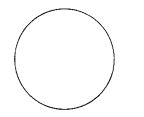(a) 1
(b) 2
(c) 3
(d) Infinitely many

Symmetry Class 7 Extra Questions Chapter 14  Question 13.
How many lines of symmetry are there in an isosceles triangle?
(a) 4
(b) 3
(c) 1
(d) 2

Symmetry MCQ Chapter 14  Question 14.
How many lines of symmetry are there in a scalene triangle?
(a) 1
(b) 0
(c) 2
(d) 4

Questions On Symmetry For Class 7 With Answers Chapter 14  Question 15.
How many lines of symmetry are there in a rhombus?
(a) 1
(b) 2
(c) 3
(d) 4

Class 7 Maths Symmetry MCQ Chapter 14  Question 16.
How many lines of symmetry are there in a parallelogram?
(a) 0
(b) 1
(c) 2
(d) None of these

MCQ Questions For Class 7 Maths Chapter 14 Question 17.
How many lines of symmetry are there in a quadrilateral?
(a) 0
(b) 2
(c) 4
(d) None of these

Questions On Symmetry For Class 7 Chapter 14  Question 18.
The order of rotational symmetry of an equilateral triangle is
(a) 1
(b) 2
(c) 3
(d) 4

Symmetry Questions For Class 7 Chapter 14  Question 19.
The order of rotational symmetry of a square is
(a) 1
(b) 2
(c) 3
(d) 4

Question 20.
What is the order of the rotational symmetry of the following figure?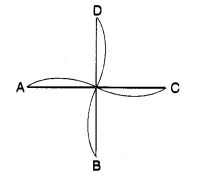(a) 4
(b) 3
(c) 2
(d) 1

Question 21.
The order of the rotational symmetry of the following figure about the point marked x (cross) is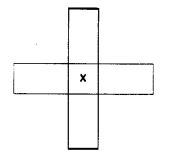(a) 1
(b) 2
(c) 3
(d) 4

Question 22.
The order of the rotational symmetry of the following figure about the point marked x is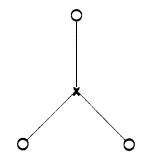(a) 1
(b) 2
(c) 3
(d) 4

Question 23.
The order of the rotational symmetry of the following figure about the point marked x is(a) 2
(b) 3
(c) 4
(d) 1

Question 24.
Which of the following letters of English alphabet has reflectional symmetry about a vertical mirror?
(a) H
(b) J
(c) Z
(d) P

Question 25.
Which of the following letters of English alphabet has reflectional symmetry about a horizontal mirror?
(a) H
(b) K
(c) M
(d) W

Question 26.
Which of the following letters of English alphabet has reflectional symmetry about both horizontal and vertical mirrors?
(a) O
(b) Y
(c) T
(d) L

Question 27.
The quadrilateral which has both line and rotational symmetry of order more than 1 is
(a) isosceles triangle
(b) rhombus
(c) scalene triangle
(d) square